# Making Sense of the Binary Logistic Regression Tool

By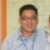Chew Jian Chieh

In some situations, Six Sigma practitioners find a Y that is discrete and Xs that are continuous. How can a regression equation be developed in these cases? Black Belt training indicated that the correct technique is something called logistic regression but this tool is often not well understood. An example about a well-known space shuttle accident can help to demystify logistic regression using the simplest logistic regression – binary logistic regression, where the Y has just two potential outcomes (i.e., “yes” or “no,” or 0 or 1).

The data in Table 1 comes from the Presidential Commission on the Space Shuttle Challenger Accident (1986). The data consists of the number of the flight, the air temperature at the time of the launch and whether or not there was damage to the booster rocket field joints (no = 0, yes = 1).

Handpicked Content:   How to Avoid Common Mistakes in Linear Regression
 Table 1: Data from Shuttle Investigation Flight Temp. Damage Flight Temp. Damage STS 1 66 STS 51A 67 STS 2 70 1 STS 51C 53 1 STS 3 69 STS 51D 67 STS 5 68 STS 51B 75 STS 6 67 STS 51G 70 STS 7 72 STS 51F 81 STS 8 73 STS 51I 76 STS 9 70 STS 51J 79 STS 41B 57 1 STS 61A 75 1 STS 41C 63 1 STS 61B 76 STS 41D 70 1 STS 61C 58 1

Using normal regression and given a particular temperature at launch time, this data can be used to calculate the probability of damage to the booster rocket field joints.

There are five steps to apply logistics regression.

### Step 1. Graphically Visualize the Data

A stratified dot plot can be used to graphically display the data. It is obvious from Figure 2 that the probability of damage is greater at lower temperatures. However, there is quite a fair bit of overlap in the distribution. Is launch temperature a real X (i.e., a real predictor of damage)? And if so, what is the probability of damage for any given launch temperature?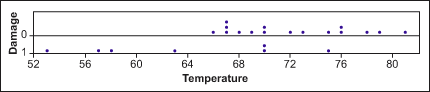Figure 1: Stratified Dot Plot

It is obvious that the probability of damage is greater at lower temperatures. However, there is quite a fair bit of overlap in the distribution. Is launch temperature a real X (i.e., a real predictor of damage)? And if so, what is the probability of damage for any given launch temperature?

Handpicked Content:   Experiential Learning of Lean Six Sigma with Marbles and Toy Cars

### Step 2. Formulate the Regression Model

Any regression requires a continuous output or Y. However, in this case the Y is discrete with only two categories or two events: Damage – yes or no. What to do? The “trick” behind the logistic regression is to turn the discrete output into a continuous output by calculating the probability (p) for the occurrence of a specific event. That means, the logistic regression provides a model to predict the p for a specific event for Y (here, the damage of booster rocket field joints, p = P[Y=1]) given any value of X (here, the temperature at the time of the launch). The logistic regression equation has the form: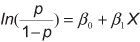This function is the so-called “logit” function where this regression has its name from. The procedure for modeling a logistic model is determining the actual percentages for an event as a function of the X and finding the best constant and coefficients fitting the different percentages.

This is exactly the equation that comes out of statistical software’s output for logistics regression: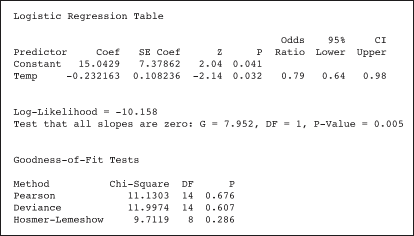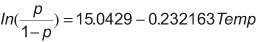### Step 3. Check Validity of Regression Model

There are two major checks that need to be done before it can said with confidence that this model is valid (please refer to the session output):

1. P-value for the coefficient is less than 0.05.
A p-value is calculated for each coefficient. If the p-value is low then there is a significant relationship between the X variable and the Y. In this case, the coefficient for temperature has p-value of 0.032 (i.e., there is a significant relationship between the temperature and the probability of a damage).
2. P-value of the “goodness of fit” tests are greater than 0.05.
Goodness-of-fit tests are conducted to see whether the model adequately fits the actual situation. Low p-values indicate a significant difference of the model from the observed data. Hence, the p-values should be above 0.05 to show that there are no significant differences between the predicted probabilities (from the model) and the observed probabilities (from the raw data). In this case, from the goodness-of-fit tests, none of them show a significant difference – the regression model is valid.
Handpicked Content:   Linear Regression: Making Sense of a Six Sigma Tool

### Step 4. Reverse the Logit Equation

This is done to obtain an answer to the question, given a particular setting of X, what is the probability of failure? Reversing this, the result is: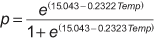On the day of the Challenger incident, the temperature was 31 degree Fahrenheit. Hence, the probability of damage to the booster rocket field joints on that day is: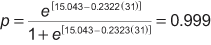Damage was almost a certainty.

### Step 5. Visualize the Results (Optional)

The event probability for all the possible temperature settings can be obtained by using statistical software. In Minitab software, for example, one must go to “Storage” and check the “Event Probability” box. The output is illustrated in Table 2.

 Table 2: Event Probability Flight Temp. Damage EPRO1 1 STS 1 66 0.430493 2 STS 2 70 1 0.229968 3 STS 3 69 0.273621 4 STS 5 68 0.322094 5 STS 6 67 0.374724 6 STS 7 72 0.158049 7 STS 8 73 0.129546 8 STS 9 70 * 9 STS 41B 57 1 0.859317

* are repetitions

Using this data, the scatter plot (decreasing logistic plot) in Figure 2 can be produced.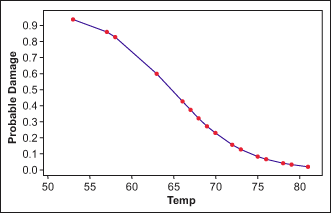Figure 2: Scatter Plot of Damage Versus Temperature

##### Chew Jian Chieh

1.Michel Lopes

Nice article. At step 4 does minitab give me that equation or I have to memorize it? It’s a very useful equation.

2.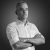Carlos Bon

Hello. I use the information of this example but I’ve reached a similar response with Minitab16. It’s not exactly the values that you presented here. I did this:

Stat > Regression > Binary Logistic Regression > Response in response/frequency format > Response: Damage > Model: Temp > Options > Reference option > Event: “0” > OK > OK

And the results are:

Response Information
Variable Value Count
Damage 0 15 (Event)
1 7
Total 22
Logistic Regression Table
Odds 95% CI
Predictor Coef SE Coef Z P Ratio Lower Upper
Constant -14,6857 7,41400 -1,98 0,048
Temp 0,226697 0,108935 2,08 0,037 1,25 1,01 1,55

Log-Likelihood = -10,110
Test that all slopes are zero: G = 7,301, DF = 1, P-Value = 0,007

Goodness-of-Fit Tests

Method Chi-Square DF P
Pearson 10,8249 13 0,625
Deviance 11,9032 13 0,536
Hosmer-Lemeshow 10,7347 7 0,151

The equation (with my results): ln (p/1-p) = – 14,6857 + 0,226697 Temp

Did I do something different?
Thank you. Regards.

3.Carlos

Hello. I use the information of this example but I’ve reached a similar response with Minitab16. It’s not exactly the values that you presented here. I did this:

Stat > Regression > Binary Logistic Regression > Response in response/frequency format > Response: Damage > Model: Temp > Options > Reference option > Event: “0” > OK > OK

And the results are:

Response Information
Variable Value Count
Damage 0 15 (Event)
1 7
Total 22
Logistic Regression Table
Odds 95% CI
Predictor Coef SE Coef Z P Ratio Lower Upper
Constant -14,6857 7,41400 -1,98 0,048
Temp 0,226697 0,108935 2,08 0,037 1,25 1,01 1,55

Log-Likelihood = -10,110
Test that all slopes are zero: G = 7,301, DF = 1, P-Value = 0,007

Goodness-of-Fit Tests

Method Chi-Square DF P
Pearson 10,8249 13 0,625
Deviance 11,9032 13 0,536
Hosmer-Lemeshow 10,7347 7 0,151

The equation (with my results): ln (p/1-p) = – 14,6857 + 0,226697 Temp

Did I do something different?
Thank you. Regards.